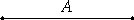# Proposition 34

If an [even] number neither is one of those which is continually doubled from a dyad, nor has its half odd, then it is both even-times-even and even-times-odd.

Let the [even] number A neither be one of those doubled from a dyad, nor have its half odd.

I say that A is both even-times-even and even-times-odd.VII.Def.8

Now that A is even-times-even is clear, for it has not its half odd.

I say next that it is also even-times-odd.

If we bisect A, then bisect its half, and do this continually, we shall come upon some odd number which measures A according to an even number. If not, we shall come upon a dyad, and A will be among those which are doubled from a dyad, which is contrary to the hypothesis.

Thus A is even-times odd.

But it was also proved even-times-even. Therefore A is both even-times-even and even-times-odd.

Therefore, if an [even] number neither is one of those which is continually doubled from a dyad, nor has its half odd, then it is both even-times-even and even-times-odd.

Q.E.D.

## Guide

Numbers which are both even-times-even and even-times-odd are are both divisible by 4 and an odd number. They are the numbers whose halves are even excluding powers of 2.

This completes the series of propositions on even and odd numbers that started with IX.21.